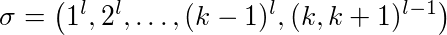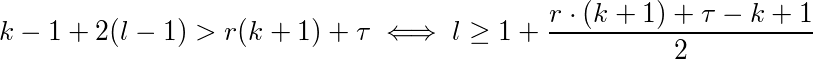# Online algorithms

suggest change

## Theory

Definition 1: An optimization problem Π consists of a set of instances ΣΠ. For every instance σ∈ΣΠ there is a set Ζσ of solutions and a objective function fσ : Ζσ → ℜ≥0 which assigns apositive real value to every solution. We say OPT(σ) is the value of an optimal solution, A(σ) is the solution of an Algorithm A for the problem Π and wA(σ)=fσ(A(σ)) its value.

Definition 2: An online algorithm A for a minimization problem Π has a competetive ratio of r ≥ 1 if there is a constant τ∈ℜ with

wA(σ) = fσ(A(σ)) ≤ r ⋅ OPT(&sigma) + τ

for all instances σ∈ΣΠ. A is called a r-competitive online algorithm. Is even

wA(σ) ≤ r ⋅ OPT(&sigma)

for all instances σ∈ΣΠ then A is called a strictly r-competitive online algorithm.

Proposition 1.3: LRU and FWF are marking algorithm.

Proof: At the beginning of each phase (except for the first one) FWF has a cache miss and cleared the cache. that means we have k empty pages. In every phase are maximal k different pages requested, so there will be now eviction during the phase. So FWF is a marking algorithm. Lets assume LRU is not a marking algorithm. Then there is an instance σ where LRU a marked page x in phase i evicted. Let σt the request in phase i where x is evicted. Since x is marked there has to be a earlier request σt* for x in the same phase, so t* < t. After t* x is the caches newest page, so to got evicted at t the sequence σt*+1,…,σt has to request at least k from x different pages. That implies the phase i has requested at least k+1 different pages which is a contradictory to the phase definition. So LRU has to be a marking algorithm.

Proposition 1.4: Every marking algorithm is strictly k-competitive.

Proof: Let σ be an instance for the paging problem and l the number of phases for σ. Is l = 1 then is every marking algorithm optimal and the optimal offline algorithm cannot be better. We assume l ≥ 2. the cost of every marking algorithm for instance σ is bounded from above with l ⋅ k because in every phase a marking algorithm cannot evict more than k pages without evicting one marked page. Now we try to show that the optimal offline algorithm evicts at least k+l-2 pages for σ, k in the first phase and at least one for every following phase except for the last one. For proof lets define l-2 disjunct subsequences of σ. Subsequence i ∈ {1,…,l-2} starts at the second position of phase i+1 and end with the first position of phase i+2. Let x be the first page of phase i+1. At the beginning of subsequence i there is page x and at most k-1 different pages in the optimal offline algorithms cache. In subsequence i are k page request different from x, so the optimal offline algorithm has to evict at least one page for every subsequence. Since at phase 1 beginning the cache is still empty, the optimal offline algorithm causes k evictions during the first phase. That shows that

wA(σ) ≤ l⋅k ≤ (k+l-2)k ≤ OPT(σ) ⋅ k

Corollary 1.5: LRU and FWF are strictly k-competitive.

Is there no constant r for which an online algorithm A is r-competitive, we call A not competitive.

Proposition 1.6: LFU and LIFO are not competitive.

Proof: Let l ≥ 2 a constant, k ≥ 2 the cache size. The different cache pages are nubered 1,…,k+1. We look at the following sequence:First page 1 is requested l times than page 2 and so one. At the end there are (l-1) alternating requests for page k and k+1. LFU and LIFO fill their cache with pages 1-k. When page k+1 is requested page k is evicted and vice versa. That means every request of subsequence (k,k+1)l-1 evicts one page. In addition their are k-1 cache misses for the first time use of pages 1-(k-1). So LFU and LIFO evict exact k-1+2(l-1) pages. Now we must show that for every constant τ∈ℜ and every constan r ≤ 1 there exists an l so thatwhich is equal toTo satisfy this inequality you just have to choose l sufficient big. So LFU and LIFO are not competetive.

Proposition 1.7: There is no r-competetive deterministic online algorithm for paging with r < k.

## Sources

### Basic Material

1. Script Online Algorithms (german), Heiko Roeglin, University Bonn
2. Page replacement algorithm

1. Online Computation and Competetive Analysis by Allan Borodin and Ran El-Yaniv

### Source Code

1. Source code for offline caching
2. Source code for adversary game